How Cheenta works to ensure student success?
Explore the Back-Story

# Triple Integral | IIT JAM 2016 | Question 15## Question 15 - Triple Integral (IIT JAM 2016)

If the triple integral over the region bounded by the planes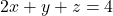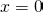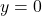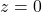is given by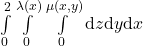then the functionis

•••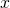•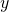### Key Concepts

Real Analysis

Integral Calculus

Triple Integral

Answer: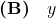IIT JAM 2016, Question No. 15

Differential and Integral Calculus: R Courant

## Try with Hints

Here we are given with triple integral over the region bounded by the planes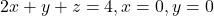andNow we our aim here is to findand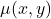. Now we will approach this problem by find the volume of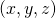based oncan you do this ??? (With the given information x=0, y=0, z=0)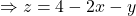[as]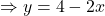Again,Now as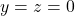we have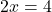Therefore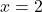Now can you use this to move forward with this problem ?

So our triple integral become,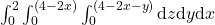On compairingand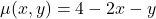Therefore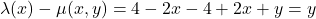(ANS)

## Question 15 - Triple Integral (IIT JAM 2016)

If the triple integral over the region bounded by the planesis given bythen the functionis

••••### Key Concepts

Real Analysis

Integral Calculus

Triple Integral

Answer:IIT JAM 2016, Question No. 15

Differential and Integral Calculus: R Courant

## Try with Hints

Here we are given with triple integral over the region bounded by the planesandNow we our aim here is to findand. Now we will approach this problem by find the volume ofbased oncan you do this ??? (With the given information x=0, y=0, z=0)[as]Again,Now aswe haveThereforeNow can you use this to move forward with this problem ?

So our triple integral become,On compairingandTherefore(ANS)

## Subscribe to Cheenta at Youtube

This site uses Akismet to reduce spam. Learn how your comment data is processed.

### Knowledge Partner